More about the examples

Next: Special cases of Up: Some planar isospectral domains Previous: Gallery of examples

# More about the examples

The examples in the previous section were obtained by applying a theorem of Sunada . Letbe a finite group. Call two subgroups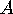and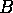ofisospectral if each element ofbelongs to just as many conjugates ofas of. (This is equivalent to requiring thatandhave the same number of elements in each conjugacy class of.) Sunada's theorem states that ifacts on a manifoldandandare isospectral subgroups of, then the quotient spaces ofbyandare isospectral.

The tables in this section show for each of the examples a trio of elements which generate the appropriate, in two distinct permutation representations. The isospectral subgroupsandare the point-stabilizers in these two permutation representations.

For the example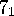, the details are as follows.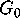is the group of motions of the hyperbolic plane generated by the reflections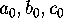in the sides of a triangle whose three angles are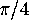. In Conway's orbifold notation (see ),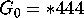.has a homomorphism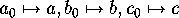onto the finite group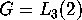(also known as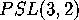), the automorphism group of the projective plane of order 2. The generators ofact on the points and lines of this plane (with respect to some unspecified numbering of the points and lines) as follows: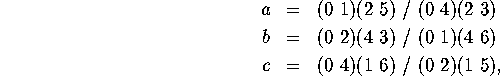where the actions on points and lines are separated by.

The grouphas two subgroupsandof index 7, namely the stabilizers of a point or a line. The preimages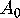andof these two groups inhave fundamental regions that consist of 7 copies of the original triangle, glued together as in Figure 2. Each of these is a hexagon of angles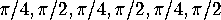, and so each ofandis a copy of the reflection group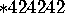.

The preimage inof the trivial subgroup ofis a groupof index 168. The quotient of the hyperbolic plane byis a 23-fold cross-surface (that is to say, the connected sum of 23 real projective planes), so that in Conway's orbifold notation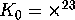. Deforming the metric on this 23-fold cross surface by replacing its hyperbolic triangles by scalene Euclidean triangles yields a cone-manifoldwhose quotients byandare non-congruent planar isospectral domains.

Tables 1 and 2 display the corresponding information for our other examples.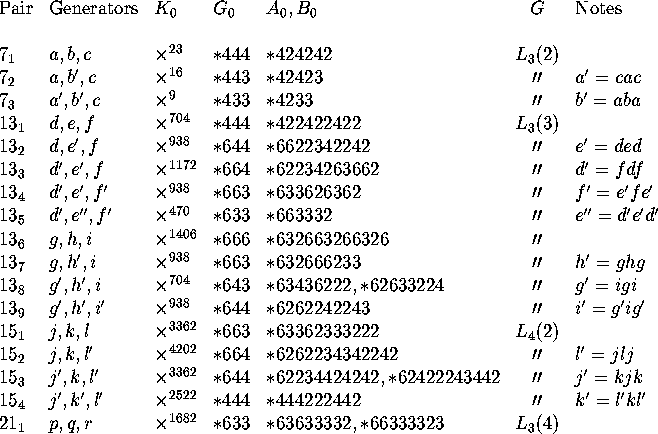Table 1: Specifications.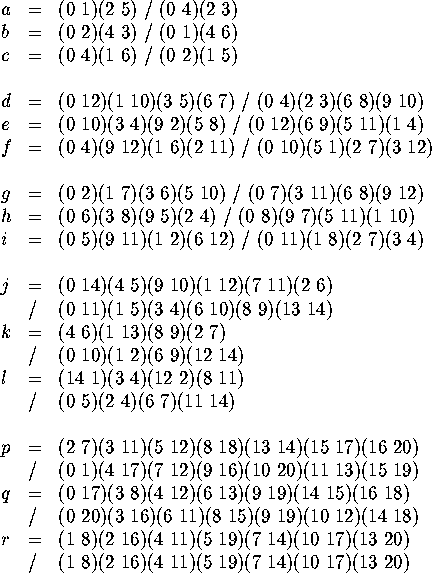Table 2: Permutations.

Note that the permutations in Table 2 correspond to the neighboring relations in Figure 4. In the propeller example, for instance, the pairs 0, 1 and 2, 5 are neighbors along a dotted line on the left-hand side, and 0, 4 and 2, 3 are neighbors along a dotted line on the right-hand side. Accordingly, we have the permutations a = (0 1)(2 5) / (0 4)(2 3), etc. Similar relations will hold in the other pairs of diagrams if the triangles are properly labelled.

Next: Special cases of Up: Some planar isospectral domains Previous: Gallery of examples

Peter Doyle